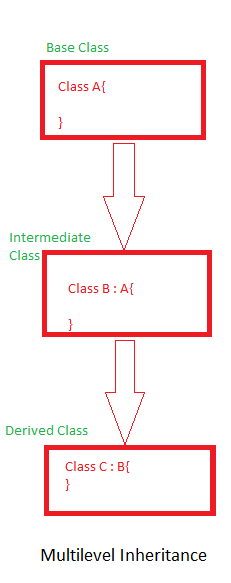# C# Inheritance

C# is a language based on Object oriented programming concepts, in last tutorial, we learned about Class and object, now, in this chapter, we will learn about inheritance in C# ( another OOP concept). Inheritance is simply process of creating one class from another class, so it allows us to use feature of one main ( base-class) into another class ( derived class). C# gives us polymorphism through inheritance.

C# doesn't support multiple inheritance.

The class which is inherited is called as base class and the class which will inherit properties and methods of base class is known as derived class.

Syntax

``````<Access_Modifier> class DerivedClass_Namee:BaseClass_Name
{
//code
}``````

Example:

``````using System;

public class Program
{
//base class, Animal
public class Animal
{

public void Talk()
{
Console.WriteLine("Animal talk");
}

};

//inherits Animal class
class Dog : Animal
{

public void Talk()
{
Console.WriteLine("Dog talk");
}

};
public static void Main()
{
Animal a1 = new Animal();
a1.Talk();

Dog a2 = new Dog();
a2.Talk();
}
}``````

Output

``````Animal talk
Dog talk``````

As you can see in the above example, we were able to override the method `Talk()` of `Animal` class into derived class `Dog`.

Now, suppose, I remove the `Talk()` method from derived class (dog)

``````using System;

public class Program
{
//base class, Animal
public class Animal
{

public void Talk()
{
Console.WriteLine("Animal talk");
}

};

//inherits Animal class
class Dog : Animal
{

};
public static void Main()
{
Animal a1 = new Animal();
a1.Talk();

Dog a2 = new Dog();
a2.Talk();
}
}``````

Output will be

``````Animal talk
Animal talk``````

Basically, now as there is no definition of `Talk()` in the derived class so simply it called the base class one.

There are 4 types of inheritance in C#

1. Single Inhertiance
2. Multilevel inhertiance
3. Hierarchical Inheritance

We just saw the example of Single Inheritance above, let's check other inheritance type examples.

### Multilevel Inheritance in C#

When a class is derived from base class than a new class inherits derived class, it is known as multi-level inheritance. Check the image below to understand itExample

``````using System;
namespace Application
{
public class A
{
public void show()
{
Console.WriteLine("Class A");
}
}
public class B : A //class B is derived by class A
{
public void display()
{
Console.WriteLine("Class B Call");
}
}
class C : B //class C is derived by class B
{
public void show1()
{
Console.WriteLine("Class C Call");
}
}
public class multilevel
{
public static void Main()
{
C obj = new C();
obj.show();               // super class member function
obj.display();                 // base class member function
obj.show1();                 // own member function
}
}

}``````

Output:

``````Class A
Class B Call
Class C Call``````

### Hierarchical Inheritance

In this type of inheritance there are multiple classes which are derived from one base class. It is used when one class feature is required in multiple classes. Let us have a look on the example:

``````using System;
namespace Application {
public class A {
public void show() {
Console.WriteLine("Show from A");
}
}
public class B: A //class B is derived by class A
{
public void display() {
Console.WriteLine("Display from B");
}
}
public class C: A //class C is also derived by class A
{
public void show1() {
Console.WriteLine("Display from C");
}
}
public class hierarchical {
public static void Main() {
B objl = new B();
C obj2 = new C();

objl.show();
objl.display();
obj2.show1();
}
}
}``````

Output

``````Show from A
Display from B
Display from C``````

### Achieving Multiple Inheritance in C#

As stated above multiple inheritance is not supported in C#, but to solve this issue, we can use Interface and derive class using it, check example to understand it more.

``````using System;
namespace Application {
// Base class Cost
public class Shape {

public void setSide(int s) {
side = s;
}
protected int side;
}
//interface
public interface Cost {
int getCost(int area);
}
// Derived class, using Interface and Base class
class square: Shape, Cost {
//methods in derived class
public int getArea() {
return (side * side);
}
public int getCost(int area) {
return area * 10;
}
}
public class SquareInheritance {
public static void Main(string[] args) {
square sq = new square();
int area;
sq.setSide(5);
area = sq.getArea();

// Print the area of the object.
Console.WriteLine("The area is: {0}", sq.getArea());
Console.WriteLine("The cost is: {0}", sq.getCost(area));

}
}
}``````

output:

``````The area is: 25
The cost is: 250``````

As you can see in the above code, we are using a "Shape" as base clas and added Interface "Cost" as second base class to create derived class "Square".